# Hypothesis Testing: An Introduction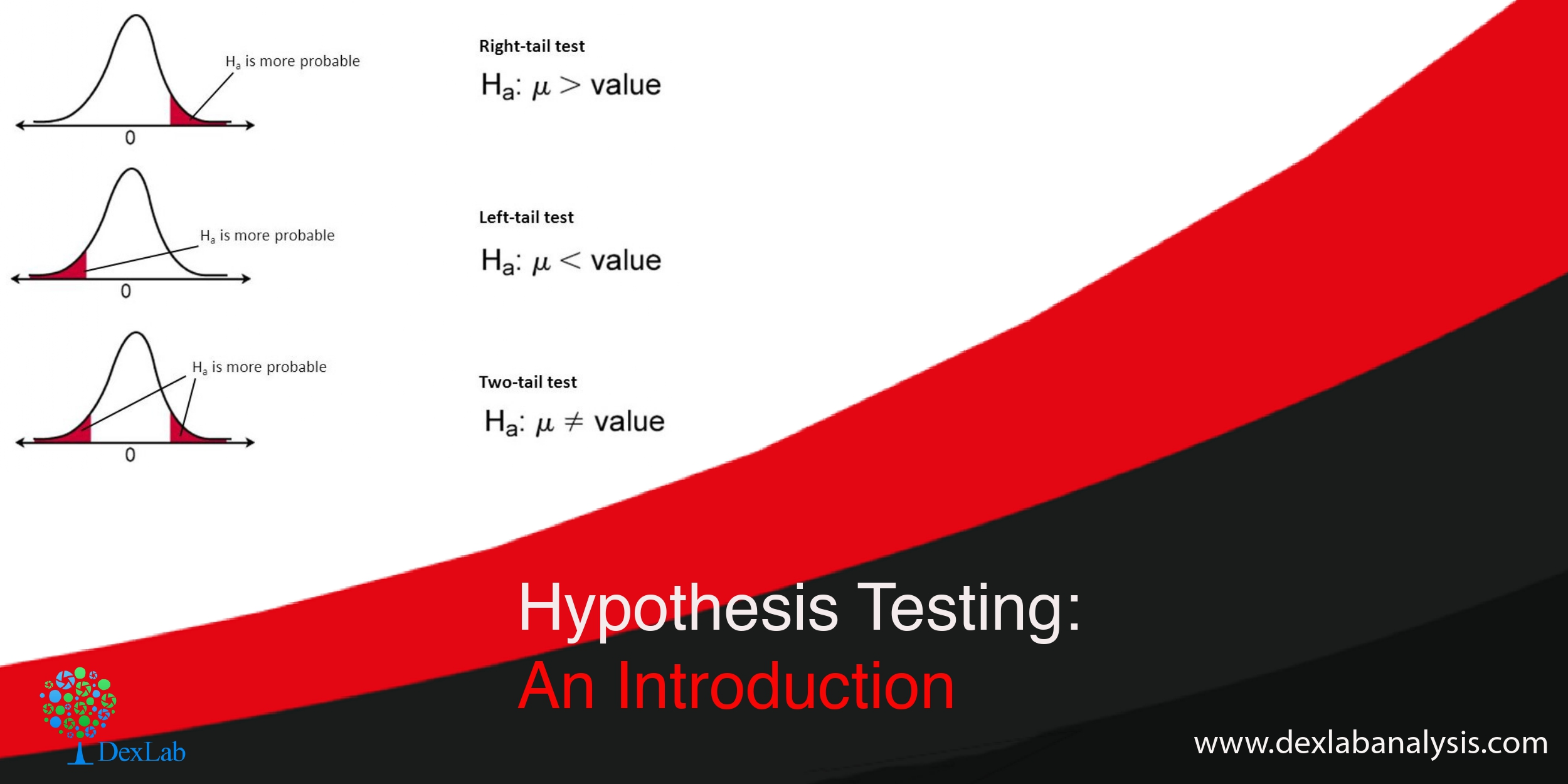You must be familiar with the phrase hypothesis testing, but, might not have a very clear notion regarding what hypothesis testing is all about. So, basically the term refers to testing a new theory against an old theory. But, you need to delve deeper to gain in-depth knowledge.

Hypothesis are tentative explanations of a principal operating in nature. Hypothesis testing is a statistical method which helps you prove or disapprove a pre-existing theory.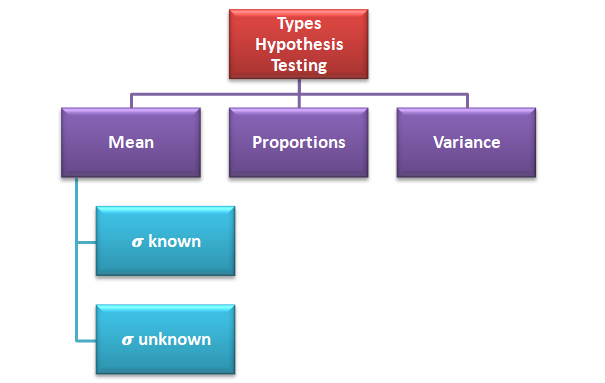Hypothesis testing can be done to check whether the average salary of all the employees has increased or not based on the previous year’s data, testing can be done to check if the percentage of passengers increased or not in the business class due to introduction of a new service and testing can also be done to check the differences in the productivity varied land.

#### There are two key concepts in testing of hypothesis:-

Null Hypothesis:- It means the old theory is correct, nothing new is happening, the system is in control, old standard is correct etc. This is the theory you want to check if is true or not. For example if a ice-cream factory owner says that their ice-cream contains 90% milk, this can be written as:-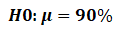Alternative Hypothesis:- It means new theory is correct, something is happening, system is out of control, there are new standards etc. This is the theory you check against the null hypothesis. For example you say that ice-cream does not contain 90% milk, this can be written as:-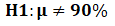#### Two-tailed, right tailed and left tailed test

Two-tailed test:- When the test can take any value greater or less than 90% in the alternative it is called two-tailed test ( H190%) i.e. you do not care if the alternative is more or less all you want to know is if it is equal to 90% or no.

Right tailed test:-When your test can take any value greater than 90% (H1>90%) in the alternative hypothesis it is called right tailed test.

Left tailed test:-When your test can take any value less than 90% (H1<90%) in the alternative hypothesis it is called left tailed test.

#### Type I error and Type II error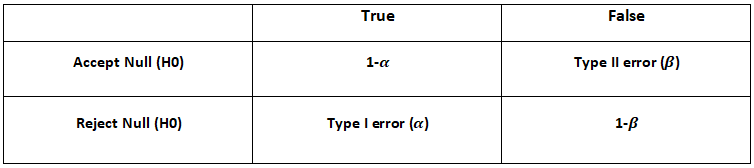->When we reject the null hypothesis when it is true we are committing type I error. It is also called significance level.

->When we accept the null hypothesis when it is false we are committing type II error.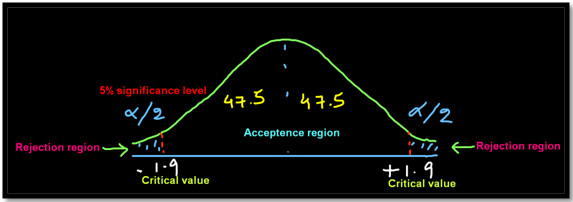#### Steps involved in hypothesis testing

1. Build a hypothesis.
2. Collect data
3. Select significance level i.e. probability of committing type I error
4. Select testing method i.e. testing of mean, proportion or variance
5. Based on the significance level find the critical value which is nothing but the value which divides the acceptance region from the rejection region
6. Based on the hypothesis build a two-tailed or one-tailed (right or left) test graph
7. Apply the statistical formula
8. Check if the statistical test falls in the acceptance region or the rejection region and then accept or reject the null hypothesis

Example:- Suppose the average annual salary of the employees in a company in 2018 was 74,914. Now you want to check whether the average salary  of the employees has increased or not in 2019. So, a sample of 112 people were taken and it was found out  that the average annual salary of the employees in 2019 is 78,795. σ=14.530.

We will apply hypothesis testing of mean when  known with 5% of significance level.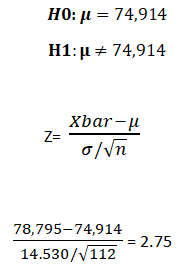The test result shows that 2.75 falls beyond the critical value of 1.9 we reject the null hypothesis which basically means that the average salary has increased significantly in 2019 compared to 2018.

So, now that we have reached at the end of the discussion, you must have grasped the fundamentals of hypothesis testing. Check out the video attached below for more information. You can find informative posts on Data Science course, on Dexlab Analytics blog.

.### Call us to know more### Gurgaon+91 931 572 5902